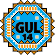General Utility Library for C++14  2.9
gul14/to_number.h

## Detailed Description

Converting strings to numbers.

## Functions

template<typename NumberType , std::enable_if_t< std::is_integral< NumberType >::value &&std::is_unsigned< NumberType >::value, int > = 0>
constexpr optional< NumberType > gul14::to_number (gul14::string_view str) noexcept
Convert an ASCII string_view into a number. More...

## ◆ to_number()

template<typename NumberType , std::enable_if_t< std::is_integral< NumberType >::value &&std::is_unsigned< NumberType >::value, int > = 0>
 constexpr optional< NumberType > gul14::to_number ( gul14::string_view str )
inlineconstexprnoexcept

Convert an ASCII string_view into a number.

This function parses the ASCII representation of a number (e.g. "123" or "1.3e10") into an optional integer or floating-point number.

gul14::optional<int> result = gul14::to_number<int>("42");
if (result.has_value())
std::cout << "The answer is " << result.value() << ".\n";
if (result)
std::cout << "The answer is " << *result << ".\n";
A class template that can either contain a value of a certain type or not.
Definition: optional.h:274

#### Design Goals

to_number() shares many characteristics with std::atoi(), std::stod() and the like, but follows its own set of design goals:

• Its input type is string_view: No null-termination and no temporary std::string are required for the conversion.
• Conversion errors are reported by returning a gul14::optional without a value.
• The function does not allocate.
• Performance should in most cases be better than and in few cases not much worse than std::sto[ildf].
• to_number<>() can be used in a constexpr context if the compiler and standard library support this. Support for floating-point numbers may lack even if integer conversion works.

#### Input Format

The allowed number format depends on the chosen numeric output type.

##### Unsigned integral types
• Accept only digits ("123", "042"=42).
##### Signed integral types
• Allow additionally a leading minus sign as well ("-42"). No leading plus sign is allowed, though.
##### Floating-point types

• A decimal point ("1.2", ".5", "12.") and exponential notation using a small or capital "e" ("12e5", "4.2e1", ".2e-4", "2.E5").
• Infinity expressions: (optional minus sign) INF or INFINITY ignoring case.
• Not-a-number expressions: (optional minus sign) NAN or NAN(char_sequence) ignoring case. The char_sequence can only contain digits, Latin letters, and underscores. The result is a quiet NaN floating-point value.

The behavior with surrounding whitespace is undefined, so it should be removed before passing input to this function. This means to_number() accepts a subset of C++17's from_chars() input format; where it supports the input format it is modeled close to from_chars().

Template Parameters
 NumberType Destination numeric type
Parameters
 str The string to be converted into a number.
Returns
a gul14::optional that contains the number if the conversion was successful. If there was a conversion error, the return value is empty. If the input describes a number whose parsed value is not in the range representable by NumberType, the return value is empty.
Note
This function has different overloads for unsigned integers, signed integers, and floating-point types.
The floating-point overload allocates an intermediate string if
• the intermediate integer type is too small in comparison to NumberType or
• this function is used with long double on Apple Clang.
Since
GUL version 1.6
GUL version 1.7 the NAN and INF floating point conversion